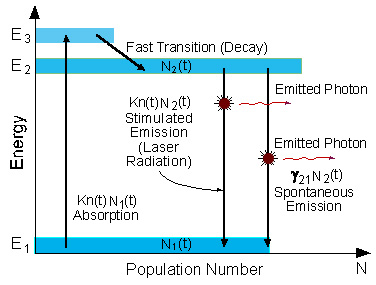Rami Arieli: "The Laser Adventure" Chapter 2.11 page 1
2.11 Three Level Laser

A schematic energy level diagram of a laser with three energy levels is shown in figure 2.6.

The two energy levels between which lasing occur are: the lower laser energy level (E1), and the upper laser energy level (E2).Figure 2.6: Energy level diagram in a three level laser

To simplify the explanation, we neglect spontaneous emission.

To achieve lasing, energy must be pumped into the system to create population inversion. So that more atoms will be in energy level E2 than in the ground level (E1).

Atoms are pumped from the ground state (E1) to energy level E3. They stay there for an average time of 10-8 [sec], and decay (usually with a non-radiative transition) to the meta-stable energy level E2.

Since the lifetime of the meta-stable energy level (E2) is relatively long (of the order of 10-3 [sec], many atoms remain in this level.

If the pumping is strong enough, then after pumping more than 50% of the atoms will be in energy level E2, a population inversion exists, and lasing can occur.

Question 2.8:
The condition of high pumping, limits the operation of a three level laser to pulsed operation. Why is continuous operation impossible in a three level laser?